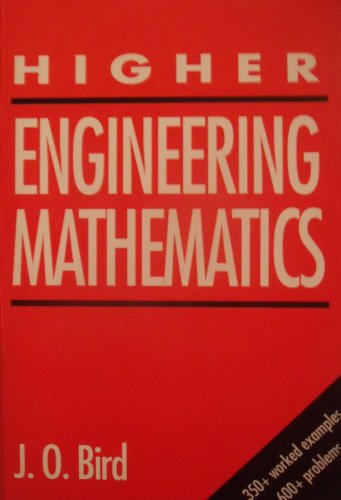# Higher Engineering Mathematics by John O. Bird

In Stock
£99.99
Dealing with the mathematical concepts and skills that are essential to engineering studies, this book is aimed at BTEC and undergraduate students. On each topic it provides a concise summary of the theory, followed by worked examples that explain how problems are solved.
Only 1 left

## Higher Engineering Mathematics Summary

### Higher Engineering Mathematics by John O. Bird

This book deals with the mathematical concepts and skills that are essential to engineering studies. On each topic it provides a concise summary of the theory, followed by worked examples that explain how problems are solved. Readers can then reinforce their understanding by working through the graded problems. This textbook caters for students on BTEC Higher National Certificate and Higher National Diploma engineering courses, and is also suitable for people embarking on engineering degree courses.

### Why buy from World of BooksOur excellent value books literally don't cost the earthFree delivery in the UKEvery used book bought is one saved from landfill

Solving equations by interactive methods; hyperbolic functions; De Moivre's theorem; the relationship between trigonometric and hyperbolic functions; methods of differentiation; differentiation of implicit functions; logarithmic differentiation; differentiation of inverse trigonometric and hyperbolic functions; partial differentiation; total differential, rates of change and small changes; introduction to integration; integration using substitutions and partial fractions; integration by parts; first order differential equations by separation of variables; homogeneous first order differential equations; linear first order differential equations; second order differential equations; fourier series for periodic functions of period 2pi; fourier series for a non-periodic function over range 2pi; even and odd functions and half-range series; fourier series over any range; a numerical method of harmonic analysis; introduction to Laplace transforms; properties of Laplace transforms; inverse Laplace transforms and solution of differential equations; linear correlation; linear regression.

GOR009973611
Higher Engineering Mathematics by John O. Bird
John O. Bird